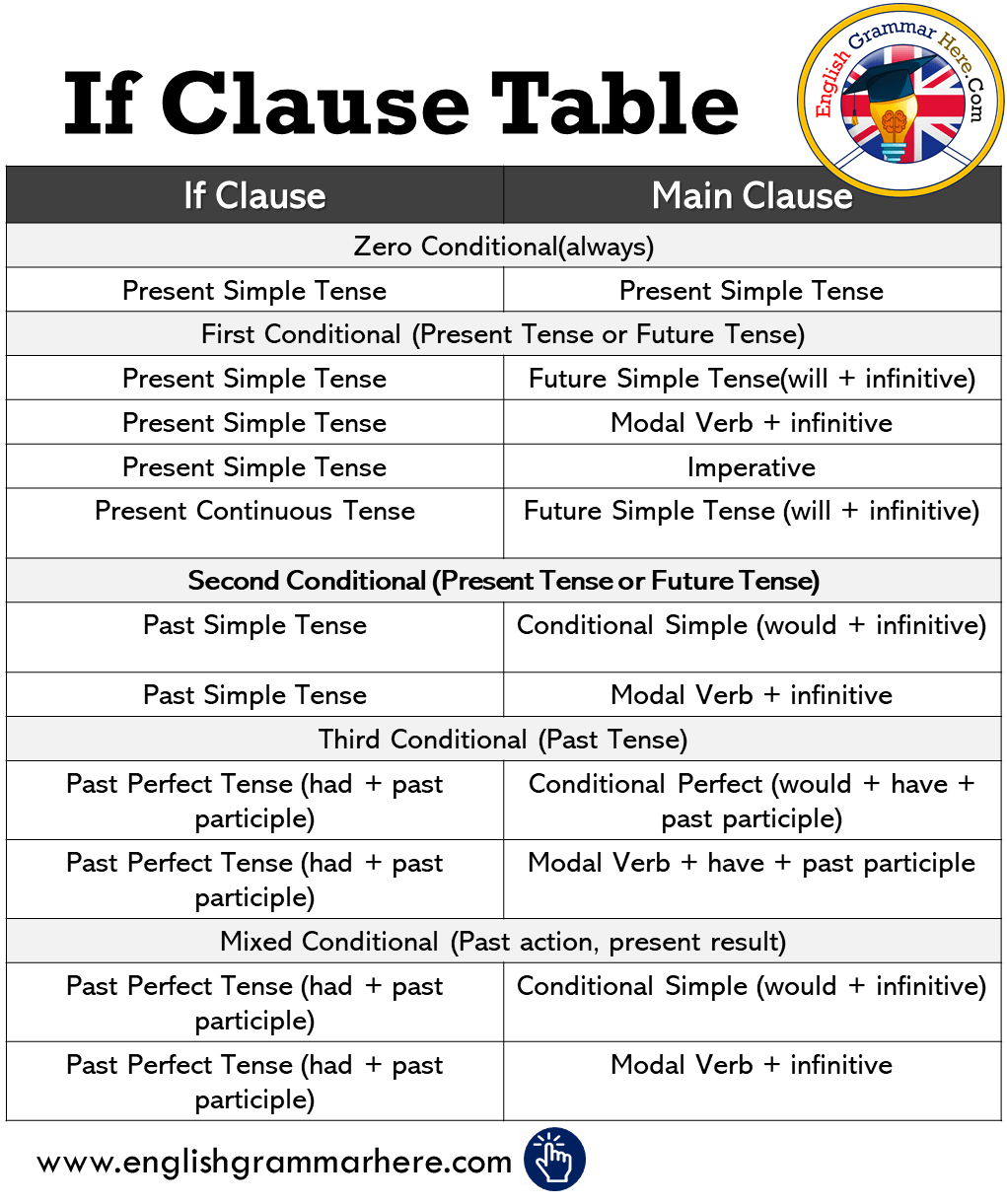# If Clause Table in English, Tenses with If Clauses

If Clause Table in English, Tenses with If ClausesIf Clause Main Clause Zero Conditional(always) Present Simple Tense Present Simple Tense First Conditional (Present Tense or Future Tense) Present Simple Tense Future Simple Tense(will + infinitive) Present Simple Tense Modal Verb + infinitive Present Simple Tense Imperative Present Continuous Tense Future Simple Tense (will + infinitive) Second Conditional (Present Tense or Future Tense) Past Simple Tense Conditional Simple (would + infinitive) Past Simple Tense Modal Verb + infinitive Third Conditional (Past Tense) Past Perfect Tense (had + past participle) Conditional Perfect (would + have + past participle) Past Perfect Tense (had + past participle) Modal Verb + have + past participle Mixed Conditional (Past action, present result) Past Perfect Tense (had + past participle) Conditional Simple (would + infinitive) Past Perfect Tense (had + past participle) Modal Verb + infinitive